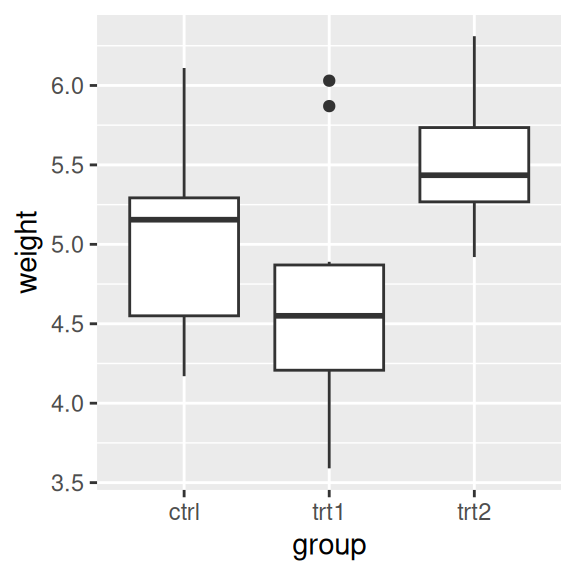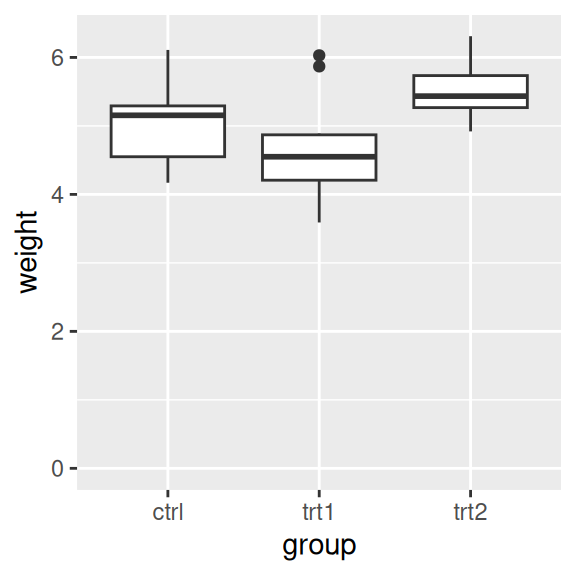## 8.2 Setting the Range of a Continuous Axis

### 8.2.1 Problem

You want to set the range (or limits) of an axis.

### 8.2.2 Solution

You can use `xlim()` or `ylim()` to set the minimum and maximum values of a continuous axis. Figure 8.3 shows one graph with the default y limits, and one with manually set y limits:

``````pg_plot <- ggplot(PlantGrowth, aes(x = group, y = weight)) +
geom_boxplot()

# Display the basic graph
pg_plot

pg_plot +
ylim(0, max(PlantGrowth\$weight))``````Figure 8.3: Box plot with default range (left); With manually set range (right)

The latter example sets the y range from 0 to the maximum value of the `weight` column, though a constant value (like 10) could instead be used as the maximum.

### 8.2.3 Discussion

`ylim()` is shorthand for setting the limits with `scale_y_continuous()`. (The same is true for `xlim()` and `scale_x_continuous()`.) The following are equivalent:

``````ylim(0, 10)
scale_y_continuous(limits = c(0, 10))``````

Sometimes you will need to set other properties of `scale_y_continuous()`, and in these cases using `xlim()` and `scale_y_continuous()` together may result in some unexpected behavior, because only the first of the directives will have an effect. In these two examples, `ylim(0, 10)` should set the y range from 0 to 10, and `scale_y_continuous(breaks=c(0, 5, 10))` should put tick marks at 0, 5, and 10. However, in both cases, only the second directive has any effect:

``````pg_plot +
ylim(0, 10) +
scale_y_continuous(breaks = NULL)

pg_plot +
scale_y_continuous(breaks = NULL) +
ylim(0, 10)``````

To make both changes work, get rid of `ylim()` and set both limits and breaks in `scale_y_continuous()`:

``````pg_plot +
scale_y_continuous(limits = c(0, 10), breaks = NULL)``````

In ggplot, there are two ways of setting the range of the axes. The first way is to modify the scale, and the second is to apply a coordinate transform. When you modify the limits of the x or y scale, any data outside of the limits is removed – that is, the out-of-range data is not only not displayed, it is removed from consideration entirely. (It will also print a warning when this happens.)

With the box plots in these examples, if you restrict the y range so that some of the original data is clipped, the box plot statistics will be computed based on clipped data, and the shape of the box plots will change.

With a coordinate transform, the data is not clipped; in essence, it zooms in or out to the specified range. Figure 8.4 shows the difference between the two methods:

``````pg_plot +
scale_y_continuous(limits = c(5, 6.5))  # Same as using ylim()
#> Warning: Removed 13 rows containing non-finite values (`stat_boxplot()`).

pg_plot +
coord_cartesian(ylim = c(5, 6.5))``````
<img src=“R-Graphics-Cookbook-2e_files/figure-html/FIG-AXES-RANGE-SCALE-COORD-1.png” alt=“Smaller y range using a scale (data has been dropped, so the box plots have changed shape; left);”Zooming in" using a coordinate transform (right)" width=“288” /><img src=“R-Graphics-Cookbook-2e_files/figure-html/FIG-AXES-RANGE-SCALE-COORD-2.png” alt=“Smaller y range using a scale (data has been dropped, so the box plots have changed shape; left);”Zooming in" using a coordinate transform (right)" width=“288” />

Figure 8.4: Smaller y range using a scale (data has been dropped, so the box plots have changed shape; left); “Zooming in” using a coordinate transform (right)

Finally, it’s also possible to expand the range in one direction, using `expand_limits()` (Figure 8.5). You can’t use this to shrink the range, however:

``````pg_plot +
expand_limits(y = 0)``````Figure 8.5: Box plot on which y range has been expanded to include 0